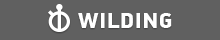GMAT Question of the Day - Daily to your Mailbox; hard ones only

 It is currently 25 Jun 2019, 19:20GMAT Club Daily Prep

Thank you for using the timer - this advanced tool can estimate your performance and suggest more practice questions. We have subscribed you to Daily Prep Questions via email.

Customized
for You

we will pick new questions that match your level based on your Timer History

Track

every week, we’ll send you an estimated GMAT score based on your performance

Practice
Pays

we will pick new questions that match your level based on your Timer History

Not interested in getting valuable practice questions and articles delivered to your email? No problem, unsubscribe here.In the figure, lines l and k are parallel. Which one of the following

Author Message
TAGS:

Hide Tags

Math ExpertV
Joined: 02 Sep 2009
Posts: 55801
In the figure, lines l and k are parallel. Which one of the following  [#permalink]

Show Tags00:00

Difficulty:45% (medium)

Question Stats:54% (01:50) correct46% (02:08) wrongbased on 28 sessions

HideShow timer StatisticsIn the figure, lines $$l$$ and $$k$$ are parallel. Which one of the following must be true?

(A) $$a < b$$

(B) $$a ≤ b$$

(C) $$a = b$$

(D) $$a ≥ b$$

(E) $$a > b$$

Attachment:#GREpracticequestion In the figure, lines l and k are parallel.jpg [ 18.37 KiB | Viewed 461 times ]

_________________
CEOP
Joined: 18 Aug 2017
Posts: 3920
Location: India
Concentration: Sustainability, Marketing
GPA: 4
WE: Marketing (Energy and Utilities)
Re: In the figure, lines l and k are parallel. Which one of the following  [#permalink]

Show Tags

Bunuel wrote:In the figure, lines $$l$$ and $$k$$ are parallel. Which one of the following must be true?

(A) $$a < b$$

(B) $$a ≤ b$$

(C) $$a = b$$

(D) $$a ≥ b$$

(E) $$a > b$$

Attachment:
#GREpracticequestion In the figure, lines l and k are parallel.jpg

solving for the given angles
we get b = 75
and a = 150
IMO E a>b
_________________
If you liked my solution then please give Kudos. Kudos encourage active discussions.
InternB
Joined: 08 Apr 2019
Posts: 19
Location: India
Schools: IIM
Re: In the figure, lines l and k are parallel. Which one of the following  [#permalink]

Show Tags

From the diagram. A=2B+30, Hence A will always be greater than B. Hence Option E
InternB
Joined: 21 Apr 2019
Posts: 1
Re: In the figure, lines l and k are parallel. Which one of the following  [#permalink]

Show Tags

How to solve the given angles?

Posted from my mobile device
ISB School ModeratorG
Joined: 08 Dec 2013
Posts: 457
Location: India
Concentration: Nonprofit, Sustainability
GMAT 1: 630 Q47 V30WE: Operations (Non-Profit and Government)
Re: In the figure, lines l and k are parallel. Which one of the following  [#permalink]

Show Tags

RupamPaul13 wrote:
From the diagram. A=2B+30, Hence A will always be greater than B. Hence Option E

Step 1. Draw a line through O parallel to the two given lines.

Now for all the given three parallel lines, interior alternate angles are equal. This gives us the equation:

a=2b+30, so a>b.

Drop a kudos if you find it helpful.
_________________
Kindly drop a '+1 Kudos' if you find this post helpful.

GMAT Math Book

-I never wanted what I gave up
I never gave up what I wanted-
ManagerB
Joined: 17 Sep 2017
Posts: 90
Re: In the figure, lines l and k are parallel. Which one of the following  [#permalink]

Show Tags

Hi, can anyone offer an explanation how did we get a= 2b+30, like why "a" is equal to the sum of the other 2 angles
ISB School ModeratorG
Joined: 08 Dec 2013
Posts: 457
Location: India
Concentration: Nonprofit, Sustainability
GMAT 1: 630 Q47 V30WE: Operations (Non-Profit and Government)
Re: In the figure, lines l and k are parallel. Which one of the following  [#permalink]

Show Tags

1
abhishek31 wrote:
Hi, can anyone offer an explanation how did we get a= 2b+30, like why "a" is equal to the sum of the other 2 angles

Interior alternate angles concept.

abhishek31

Attachment:IMG_20190525_180058.jpg [ 2.07 MiB | Viewed 111 times ]

_________________
Kindly drop a '+1 Kudos' if you find this post helpful.

GMAT Math Book

-I never wanted what I gave up
I never gave up what I wanted-Re: In the figure, lines l and k are parallel. Which one of the following   [#permalink] 25 May 2019, 05:32
Display posts from previous: Sort by

In the figure, lines l and k are parallel. Which one of the following